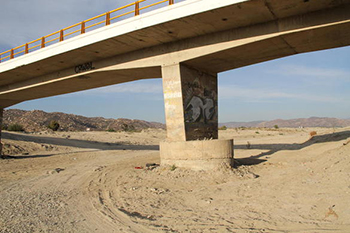online_scour_hec18:  Calculation of bridge scour using the HEC-18 formulaFormula:

Fr1 = v1/(g y1)1/2

ys /y1 = 2 K1 K2 K3 (a / y1)0.65 Fr10.43

[ SI Units (metric):  ys, y1, a, and L in m ]
[ U.S. Customary Units:  ys, y1, a, and L in ft ]
[ Fr = Froude number; g = gravitational acceleration ]

Reference:

 SAMPLE INPUT      [Main page]     [Description] To determine the bridge scour depth ys, the calculator requires the following information: Flow depth directly upstream of the pier y1 =  5.334 m Mean flow velocity directly upstream of the pier v1 =  7.15 m/s Pier width in a horizontal direction normal to the flow a =  4.52 m Pier length L =  5.1 m Angle of attack θ of the flow, θ =  0° Pier nose shape, to determine the correction factor K1  ⇒  Circular cylinder The bed condition, to determine the correction factor K3  ⇒  Clear-water scour SAMPLE OUTPUT               Scour depth ys =  10.484 m